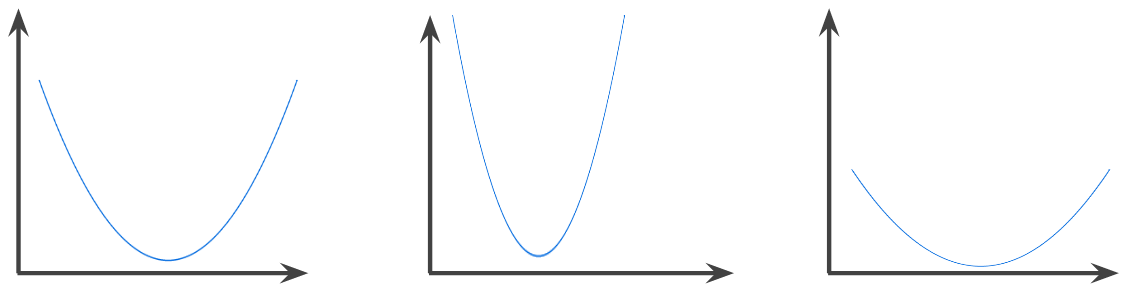# What is convex function in Machine Learning

A function in which the region above the graph of the function is a convex set. The prototypical convex function is shaped something like the letter U. For example, the following are all convex functions:By contrast, the following function is not convex. Notice how the region above the graph is not a convex set:strictly convex function has exactly one local minimum point, which is also the global minimum point. The classic U-shaped functions are strictly convex functions. However, some convex functions (for example, straight lines) are not U-shaped.

A lot of the common loss functions, including the following, are convex functions:

• L2 loss
• Log Loss
• L1 regularization
• L2 regularization

Many variations of gradient descent are guaranteed to find a point close to the minimum of a strictly convex function. Similarly, many variations of stochastic gradient descent have a high probability (though, not a guarantee) of finding a point close to the minimum of a strictly convex function.

The sum of two convex functions (for example, L2 loss + L1 regularization) is a convex function.

Deep models are never convex functions. Remarkably, algorithms designed for convex optimization tend to find reasonably good solutions on deep networks anyway, even though those solutions are not guaranteed to be a global minimum.#### Data Science PR

This site uses Akismet to reduce spam. Learn how your comment data is processed.

#### Recent posts

###### Hedera Hashgraph Price Analysis 2021 – HBAR Token Price Forecast 2021 -Hbar Price Prediction 2021Don't be shy, get in touch. We love meeting interesting people and making new friends.